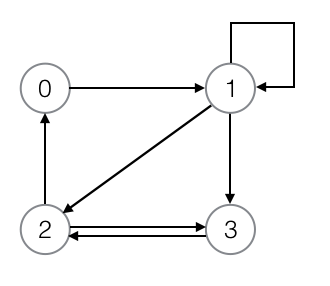Graph

The graph that we will consider can be both a directed graph and a non directed graph and can also contain cycles.

In BFS or Breadth First Search, like DFS - Depth First Search we have to keep track of vertices that are visited in order to prevent revisiting them. For this we use an array to mark visited and unvisited vertices. In BFS we also take help of a QUEUE. When a vertex is visited, we enqueue the vertex to the queue. The process ends when the queue becomes empty.

We start the process by considering any one of the vertex as the starting vertex.

### Problem

Consider the following graph.There are 4 vertices in the graph so we will need an adjacency matrix having 4 rows and 4 columns.

 Adj 0 1 2 3 0 1 2 3

Row and Column name is same as the vertex name. The cells of the adjacency matrix Adj will contain 1 if there is an edge from starting vertex to ending vertex. If there is no edge then it will contain 0.

As per the given graph our adjacency matrix will look like the following.

 Adj 0 1 2 3 0 0 1 0 0 1 0 1 1 1 2 1 0 0 1 3 0 0 1 0

To keep track of the visited vertices we will use the visited[] array. The size of this array will be equal to the number of vertices in the graph. In this case it is 4.

Initially, we will set all the elements in the array visited[] as 0 which means unvisited. As we progress we will be visiting new vertices so, we will be marking the respective index in the visited[] array with 1. Note, the vertices in the graph are names from 0 to 3 so, we can use the visited[] array index to represent the respective vertex.

We will also use a queue to enqueue and dequeue vertices into and out of it as we progress.

### Steps

Step 1: We consider a vertex as the starting vertex, in this case vertex 2.
Step 2: We enqueue vertex 2 in the queue.
Step 3: We set visited = 1 which means we have visited vertex 2.
Step 4: Print starting vertex 2 as the first result.
Step 5: If the queue is not empty then, dequeue the first vertex in the stack. Else STOP.
Step 6: Set i = dequeue vertex from the queue.
Step 7: If visited[j] == 0 AND Adj[i][j] == 1 where j = 0 to 3, then Next result is j
Step 8: Set visited[j] = 1.
Step 9: Enqueue j in the queue.
Step 10: If j reaches the last index 3 go to step 5.

### Code

``````
#include <stdio.h>

#define QUEUE_SIZE 20
#define MAX 20

//queue
int queue[QUEUE_SIZE];
int queue_front, queue_end;
void enqueue(int v);
int dequeue();

void bfs(int Adj[][MAX], int n, int source);

int main(void) {
{0,1,0,0},
{0,1,1,1},
{1,0,0,1},
{0,0,1,0}
};

int n = 4;  //no. of vertex
int starting_vertex = 2;

return 0;
}

void bfs(int Adj[][MAX], int n, int source) {
//variables
int i, j;

//visited array to flag the vertex that
//were visited
int visited[MAX];

//queue
queue_front = 0;
queue_end = 0;

//set visited for all vertex to 0 (means unvisited)
for(i = 0; i < MAX; i++) {
visited[i] = 0;
}

//mark the visited source
visited[source] = 1;

//enqueue visited vertex
enqueue(source);

//print the vertex as result
printf("%d ", source);

//continue till queue is not empty
while(queue_front <= queue_end) {
//dequeue first element from the queue
i = dequeue();

for(j = 0; j < n; j++) {
if(visited[j] == 0 && Adj[i][j] == 1) {
//mark vertex as visited
visited[j] = 1;

//push vertex into stack
enqueue(j);

//print the vertex as result
printf("%d ", j);
}
}
}
printf("\n");
}

void enqueue(int v) {
queue[queue_end] = v;
queue_end++;
}

int dequeue() {
int index = queue_front;
queue_front++;
return queue[index];
}
``````

Output: 2 0 3 1

### Time complexity

The time complexity of Breadth First Search (BFS) is O(V+E) where, V is the total number of vertices in the graph and E is the total number of edges in the graph.